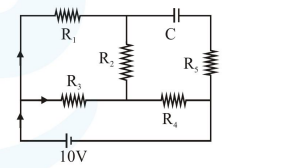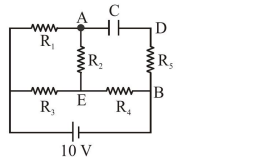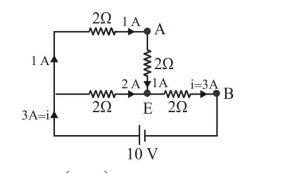# Solve this following

Question:

An ideal cell of emf $10 \mathrm{~V}$ is connected in circuit shown in figure. Each resistance is $2 \Omega$. The potential difference (in V) across the capacitor when it is fully charged isSolution:- $\mathrm{R}_{1}$ to $\mathrm{R}_{5} \rightarrow$ each $2 \Omega$

- Cap. is fully charged

- So no current is there in branch ADB

- Effective circuit of current flow :$\mathrm{R}_{e q}=\left(\frac{4 \times 2}{4+2}\right)+2$

$\mathrm{R}_{e q}=\frac{4}{3}+2=\frac{10}{3} \Omega$

$\mathrm{i}=\frac{10}{10 / 3}=3 \mathrm{~A}$

So potential different across $\mathrm{AEB}$

$\Rightarrow 2 \times 1+2 \times 3=8 \mathrm{~V}$

Hence potential difference across

Capacitor $=\Delta \mathrm{V}=\mathrm{V}_{\mathrm{AEB}}=8 \mathrm{~V}$